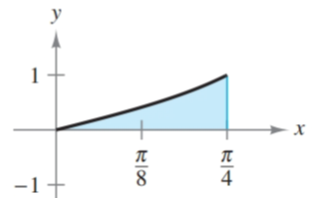Chapter 8.5, Problem 48E### Calculus: An Applied Approach (Min...

10th Edition
Ron Larson
ISBN: 9781305860919

#### Solutions

Chapter
Section### Calculus: An Applied Approach (Min...

10th Edition
Ron Larson
ISBN: 9781305860919
Textbook Problem
1 views

# Finding Area by Integration In Exercises 47-52, find the area of the region. See Example 7. y = tan xTo determine

To calculate: The area bounded by the graph of y=tanx.

Explanation

Given Information:

The provided graph of y=tanx can be shown as,

Formula used:

The formula of definite integral of abtanudu.

abtanudu=[ln|cosu|]ab

Calculation:

From provided diagram, the interval [a,b] will be [0, π4]. Therefore, area bounded by graph of y=tanx can be calculated as,

0π/4tanxdx

Integrate with the help of abtanudu formula

### Still sussing out bartleby?

Check out a sample textbook solution.

See a sample solution

#### The Solution to Your Study Problems

Bartleby provides explanations to thousands of textbook problems written by our experts, many with advanced degrees!

Get Started

#### Evaluate the limit, if it exists. limt3t292t2+7t+3

Single Variable Calculus: Early Transcendentals, Volume I

#### In problems 1-16, solve each equation. 6. Solve

Mathematical Applications for the Management, Life, and Social Sciences

#### True or False: converges.

Study Guide for Stewart's Multivariable Calculus, 8th

#### Simplify: a2aa+2a22aa24(a0,2,2)

College Algebra (MindTap Course List)